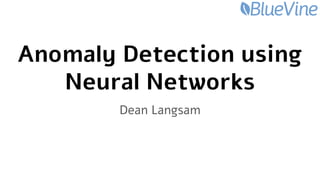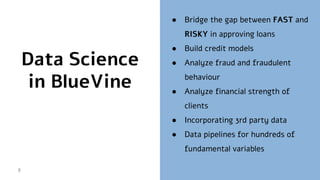Successfully reported this slideshow.

# Anomaly Detection using Neural Networks with Pandas, Keras and Python×

# Anomaly Detection using Neural Networks with Pandas, Keras and Python

@ PyData Israel 2018
● Anomaly detection project @ BlueVine
● My first neural network
● Useful and modern Pandas
● Python is a friend, not a foe.

@ PyData Israel 2018
● Anomaly detection project @ BlueVine
● My first neural network
● Useful and modern Pandas
● Python is a friend, not a foe.

### Anomaly Detection using Neural Networks with Pandas, Keras and Python

1. 1. Anomaly Detection using Neural Networks Dean Langsam
2. 2. What We Do 2 ● Mission ○ Offer convenient and flexible access to working capital for small and medium sized businesses ● Products: ○ Revolving line of credit ○ Invoice Factoring (Receivables backed financing)
3. 3. Data Science in BlueVine ● Bridge the gap between FAST and RISKY in approving loans ● Build credit models ● Analyze fraud and fraudulent behaviour ● Analyze financial strength of clients ● Incorporating 3rd party data ● Data pipelines for hundreds of fundamental variables 3
4. 4. Data Flow 4 Bank Data FICO score Industry Credit report Transactions Deals Data Internal model Credit report score BV Score Industry models Deal Score ● Several primary data sources ● Dozens of models ● Hundreds of variables ● Thousands of total "things" to monitor
5. 5. Anomaly Detection ● Many data sources ● Big Team: Each model depends on results of other models ● Model scores are mission critical for the company ● Anomalies are proxies for corrupted data 5 Why we need it?
6. 6. In this Talk 6 ● Anomaly detection project ○ My choices ○ Build it end to end ● My first neural network ● Useful and modern Pandas ● Python is a friend, not a foe.
7. 7. Initialization ● Explore: A small subset of variables ● Understanding: monitor counts data ● Realization: ○ Complex data pipeline ○ Several different underlying tasks ○ Independent tasks ● Solution: “Microservice” architecture 7 Same variable counts data on different intervals
8. 8. Pandas and datetimes ● Penny drop moments (choir moments) ● Why not ● Index with date (df.loc[]) ● df.resample() ● df.assign (col = lambda…) 8 rs = (df .set_index('date_') .dropna() .loc['2017-1':,:] .resample('d') .apply('count') .rename(columns={'var_id':'counts'}) .loc[:,['counts']] .assign(weekend= lambda df: df.index.weekday >=5) .assign(diff_ = lambda df: df.counts.diff(1)) ) rs = (df .set_index('date_') .dropna() .loc['2017-1':,:] .resample('d') .apply('count') .rename(columns={'var_id':'counts'}) .loc[:,['counts']] # .assign(weekend= lambda df: df.index.weekday >=5) .assign(diff_ = lambda df: df.counts.diff(1)) ) x = (1,2,3, 4, 5,6 ) df2 = (df .groupby('col') .count() )
9. 9. ● .dt accessor ○ df.col.dt.day ○ .floor(‘1h’) and .ceil(‘3d’) ● df.col.rolling(window).mean() ● df.col.rolling(window).std() ● df.col.expanding... ● df.col.ewm... Pandas date index 9
10. 10. Packages ● Luminol by LinkedIn ● Donut ○ Uses Tensorflow ● Skyline by Esty ○ No longer maintained 10
11. 11. Update 11 Predict Fit (Training) Alert
12. 12. 12 Update Predict Fit (Training) Alert ● Acts as a “sensor” ● Aggregates raw data into fixed-time, fixed-scope observations ● Reads production tables on fixed time-intervals and writes aggregated summaries
13. 13. 13 Update Predict Fit (Training) Alert ● Some variables write more frequently than others ● Some variables show different patterns than others
14. 14. 14 Update Predict Fit (Training) Alert ● df.pipe() ● pd.date_range() ● pd.reindex() def pad_vector_counts (df): ret = df.copy() date_index = pd.date_range(start=min_date, end=max_date, freq='H', tz='UTC', name='date_') ... ret = ret.reindex(index=date_index.union(ret.index), columns=var_ids) return ret df = (df .pipe(count_db_writes, freq='1h') .pipe(pad_db_counts,min_date=min_date, max_date=max_date,ids=ids) .pipe(expand_db_counts) )
15. 15. 1515 Update Predict Fit (Training and Retraining) Alert ● Given aggregated data, creates many time series ● Processes time-series into sliding windows ● Train/test split ● Data cleaning pipeline TestTrain
16. 16. 1616 Update Predict Fit (Training and Retraining) Alert ● Make u-turns: Use asserts ● One-stop-shop for cleaning ○ .pipe(clean) def retrain_model(data): assert complete_counts_history(data), 'meaningful message {}'.format('') # ... train_start, train_end, test_start, test_end = get_train_test_period() train_data = create_sliding_windows(data, start=train_start, end=train_end, training=True) test_data = create_sliding_windows(data, start=test_start, end=test_end, training=False) scaler = MinMaxScaler().fit(train_data[['y']]) X_train, y_train = process_input(train_data, scaler) X_test, y_test = process_input(test_data, scaler) # … return model
17. 17. 1717 Update Predict Fit (Training and Retraining) Alert ● Learns a fully-connected Neural network for each variable on counts data ● Optimizes MAE ● A good trade off between practical and efficient
18. 18. 1818 Update Predict Fit (Training and Retraining) Alert ● Create model inside a function ● Use functools.partial to iterate over options def make_dense_model(X_train, y_train, nb=16, loss='mse', dropout=0.2, optimizer='adam'): in_shp = X_train.shape out_shp = y_train.shape model = Sequential() model.add(Reshape((-1,), input_shape=(in_shp, in_shp))) model.add(Dense(2 * nb, activation='tanh')) model.add(Dropout(dropout)) model.add(Dense(nb, activation='tanh')) model.add(Dropout(dropout)) model.add(Dense(out_shp)) model.add(Activation('relu')) model.add(Reshape((out_shp, 1))) model.compile(optimizer=optimizer, loss=loss) return model from functools import partial make_model = partial(make_dense_model, nb_nodes=16, loss='mae') ### models = [ partial(make_dense_model, nb=16, loss='mae'), partial(make_lstm_model, nb=16, loss='mse'), …, partial(make_lstm_model, nb=4, loss='mse') ] ### def make_and_fit(X,y,make_model): model = make_model(X, y) # Do more things fit_model(model, X, y) return model
19. 19. 1919 Update Predict Fit (Training and Retraining) Alert ● Uses test errors to compare model to previous models and chooses the best one ● Looks at errors of individual predictions
20. 20. 2020 Update Predict Fit (Training and Retraining) Alert ● On the test period, creates a histogram of prediction error per observation ● Fits the histogram to a non-central T distribution ● Finds thresholds that constitute anomalies Progression of Errors
21. 21. 2121 Update Predict Fit (Training and Retraining) Alert ● Distribution parameters and thresholds are saved to metadata table ● Models are saved to S3 ● Model info is saved to metadata table Distribution of Errors
22. 22. 222222 Update Predict Fit (Training) Alert ● Counts data is written ● Predicted data is compared against real data ● Error is compared against thresholds ● Error and anomaly info are saved to database New Observation Error
23. 23. 232323 Update Predict Fit (Training) Alert ● Counts data is written ● Predicted data is compared against real data ● Error is compared against thresholds ● Error and anomaly info are saved to database Error
24. 24. 242424 Update Predict Fit (Training) Alert ● Luckily we have the pipeline ● All model parameters exist ○ But not as python objects ● Make sure you can recreate everything from metadata ○ even the charts ● Exception: The model itself ● Make sure you can recreate the model itself from metadata ● getattr() scaler = MinMaxScaler().fit([[db['scaler_min']], [db['scaler_max']]]) observation = create_sliding_windows(data, **db) logger.info('Sliding windows created') X, y = process_input(observation, scaler) model = load_keras_model(**db) def get_distribution_from_dict(db): dist_params = get_dist_params_from_db(db) dist = getattr(scipy.stats, db['dist_name']) return dist(*dist_params)
25. 25. 25252525 Update Predict Fit (Training) Alert ● Take all anomalies from the database ● Create chart for each anomaly ● The chart captures all relevant information for current anomaly ○ Time series of actual vs. predicted ○ Time series of error progression ○ Histogram of errors with underlying distribution
26. 26. 26262626 Update Predict Fit (Training) Alert ● Support several types of anomalies ● Feedback mechanism will allow supervised learning in the future
27. 27. 27
28. 28. 28
29. 29. 29
30. 30. 30 ● You don’t need to have a PHD in machine vision to deploy neural networks ● Pure Python has a lot more to offer than you use (But not dataframes) ● Diving into Pandas is a lot faster than inventing Pandas Conclusions
31. 31. Thank you DeanLangsam Dean_La DeanLa DeanLa.com 31